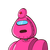# A share of FV ₹ 10 is purchased for MV ₹ 12 . Dividend declared is 18%. Complete the following activity to find the rate of return

A share of FV ₹ 10 is purchased for MV ₹ 12 . Dividend declared is 18%. Complete the following activity to find the rate of return.
Solution. ​

### 1 thought on “A share of FV ₹ 10 is purchased for MV ₹ 12 . Dividend declared is 18%. Complete the following activity to find the rate of return”

1.$$\large\underline{\sf{Given- }}$$

• Face value of share = ₹ 10
• Market value of share = ₹ 12
• Dividend %  = 18 %

$$\large\underline{\sf{To\:Find – }}$$

• Rate of return

$$\large\underline{\sf{Solution-}}$$

Given that

• Face value of share = ₹ 10
• Market value of share = ₹ 12
• Dividend %  = 18 %

Let number of shares purchased be ‘x’.

We know,

$$\rm :\longmapsto\:Income \: = \: D \: \% × \: F.\: V. \times number \: of \: shares$$

where,

• D % = Dividend %
• F. V. = Face Value of 1 share

$$\rm :\implies\:Income = \dfrac{18}{100} \times 10 \times x = 1.8x$$

Now,

• Investment = Market Value × number of shares
• Investment = ₹ 12x

So,

$$\rm :\longmapsto\:Rate \: of \: return = \dfrac{Income}{Investment} \times 100\%$$

$$\rm :\longmapsto\:Rate \: of \: return = \dfrac{1.8x}{12x} \times 100\%$$

$$\rm :\longmapsto\:Rate \: of \: return = 15\%$$

### Basic Concepts :-

Nominal value (N.V.) or face value (F.V.): The original value of a share printed in the certificate of the share is called its nominal value (N.V.) or face value (F.V.) or per value. The face value or nominal value of a share always remains same.

Market value (M.V.): The price at which the share is sold or purchased in the capital market through stock exchanges is called its market value (M.V.). The market value of a share changes from time to time.

At par: If the market value of a share is the same as its face value, the share is called at par.

At premium or above par: The share of a company that is doing well or expected to do well is sold in the market at a price higher than its face value (F.V.). In such a situation, we say the share is at a premium or above per. For example, if a share of face value of ₹ 50 is selling at ₹ 70, then it is said to be selling at a premium of ₹ 20 or at ₹ 20 above par.

At discount or below par: The share of a company that is doing poorly or may do poorly is the future is sold in the market at a price lower than its face value (F.V.). In such a situation, we say the share is at a discount or below par. For example, , if a share of face value of ₹ 50 is selling at ₹ 40, then it is said to be selling at a discount of ₹ 10 or at ₹ 10 below par.

Dividend: The profit which a share holder gets (out of the profits of the company) for his investment from the company is called dividend.